# EU Long-Term Interest Rates, October 2020

Dec 8, 2020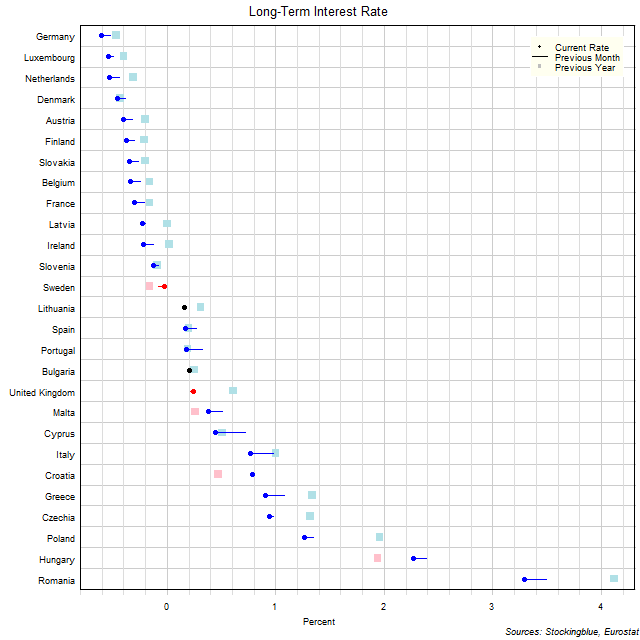The chart above shows the ten-year interest rate in each EU state as of October 2020, the change from the previous month, and the rate one year prior.  Thirteen states have a negative interest rate (same as 13 last month and up from 11 last year).

# EU Long-Term Interest Rates, September 2020

Nov 10, 2020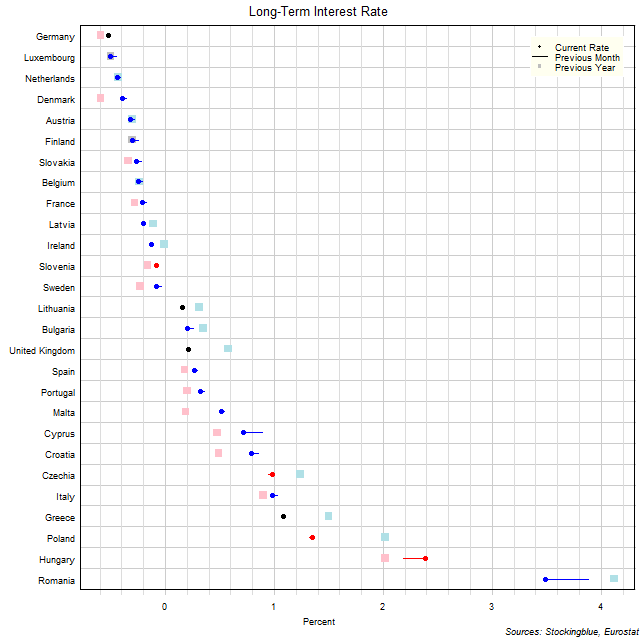The chart above shows the ten-year interest rate in each EU state as of September 2020, the change from the previous month, and the rate one year prior.  Thirteen states have a negative interest rate (same as 13 last month and same as 13 last year).

# EU Long-Term Interest Rates, August 2020

Oct 5, 2020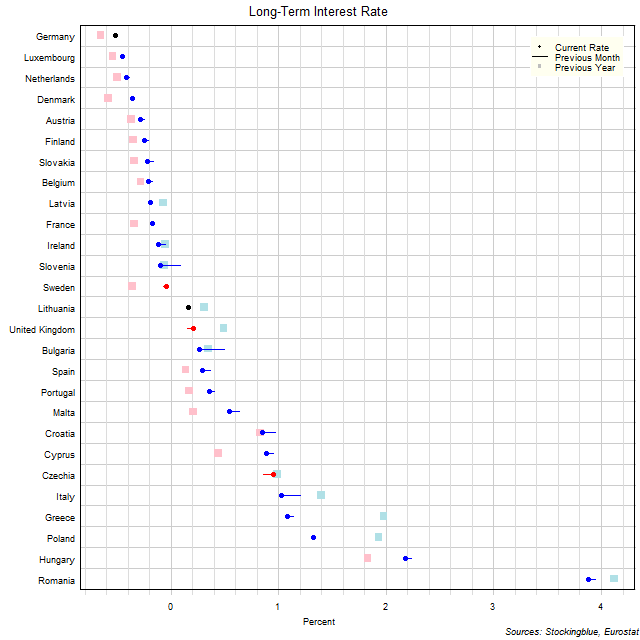The chart above shows the ten-year interest rate in each EU state as of August 2020, the change from the previous month, and the rate one year prior.  Thirteen states have a negative interest rate (up from 12 last month and same as 13 last year).

# EU Long-Term Interest Rates, July 2020

Sep 7, 2020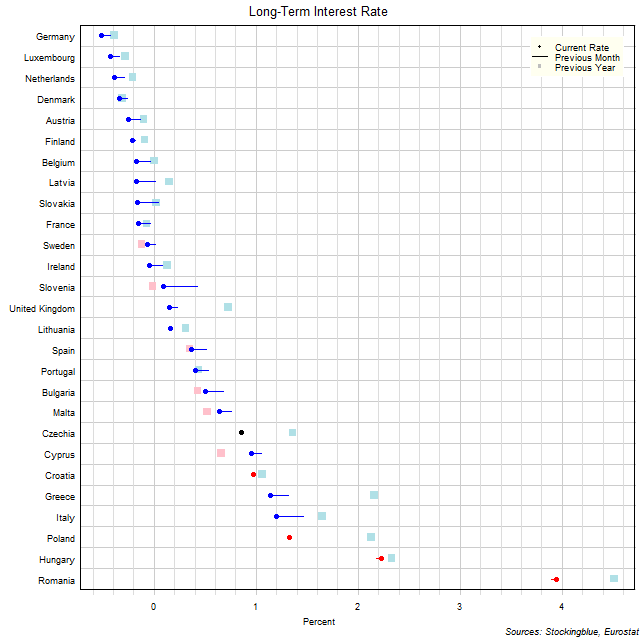The chart above shows the ten-year interest rate in each EU state as of July 2020, the change from the previous month, and the rate one year prior.  Twelve states have a negative interest rate (up from eight last month and up from nine last year).

# EU Long-Term Interest Rates, June 2020

Aug 10, 2020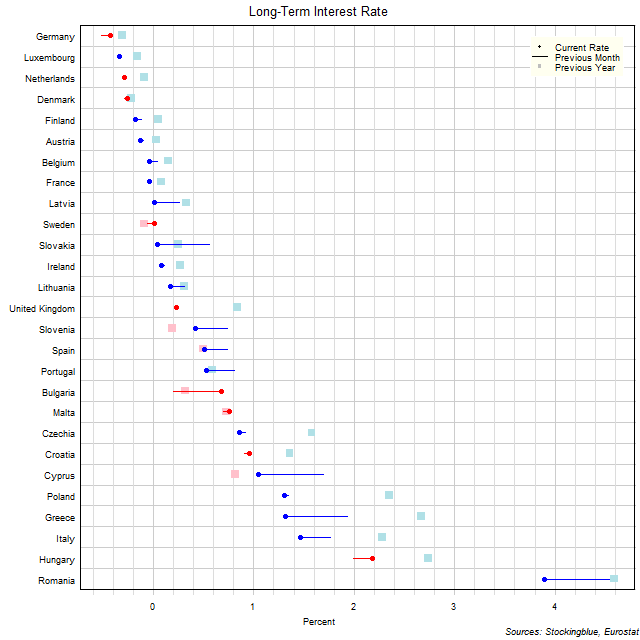The chart above shows the ten-year interest rate in each EU state as of June 2020, the change from the previous month, and the rate one year prior.  Eight states have a negative interest rate (same as eight last month and up from five last year).

# EU Long-Term Interest Rates, May 2020

Jul 3, 2020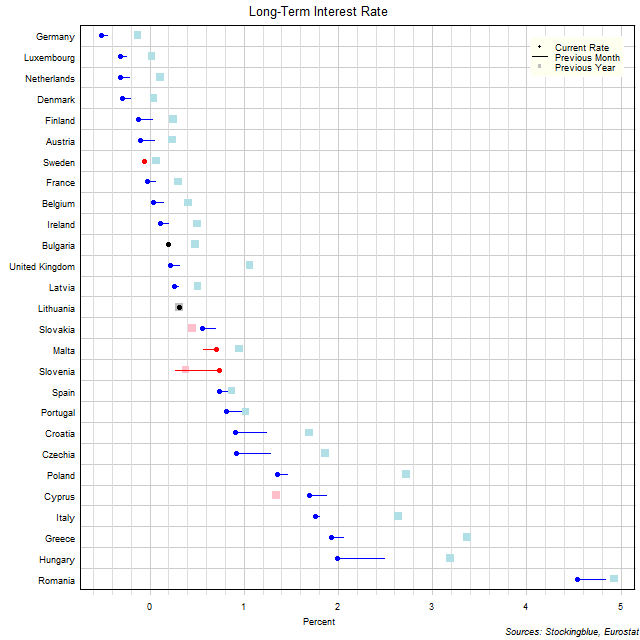The chart above shows the ten-year interest rate in each EU state as of May 2020, the change from the previous month, and the rate one year prior.  Eight states have a negative interest rate (up from five last month and up from one last year).

# EU Long-Term Interest Rates, April 2020

Jun 5, 2020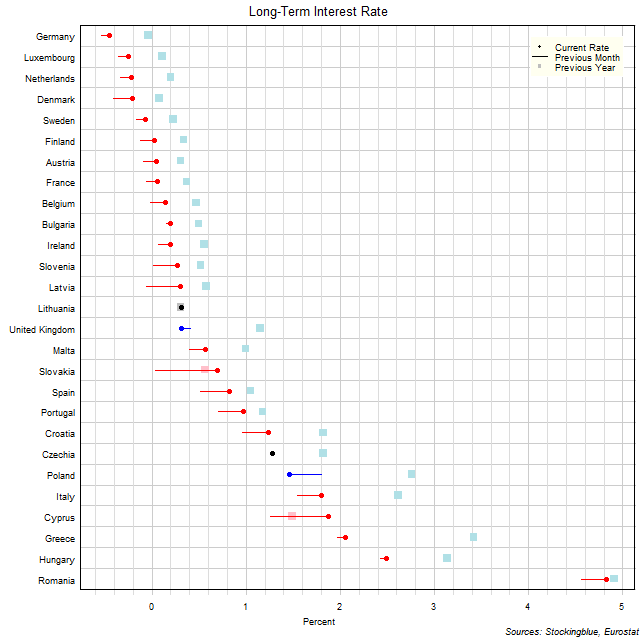The chart above shows the ten-year interest rate in each EU state as of April 2020, the change from the previous month, and the rate one year prior.  Five states have a negative interest rate (down from ten last month and up from one last year).

# EU Long-Term Interest Rates, March 2020

May 8, 2020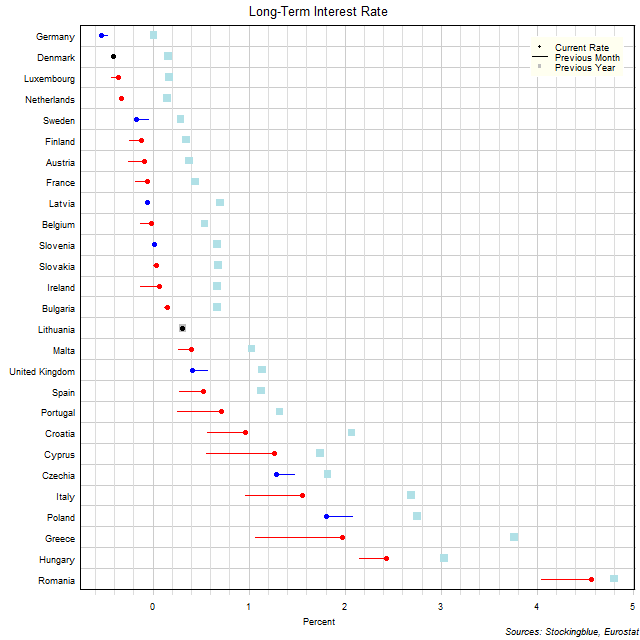The chart above shows the ten-year interest rate in each EU state as of March 2020, the change from the previous month, and the rate one year prior.  Ten states have a negative interest rate (down from eleven last month and up from zero last year).

# EU Long-Term Interest Rates, February 2020

Apr 7, 2020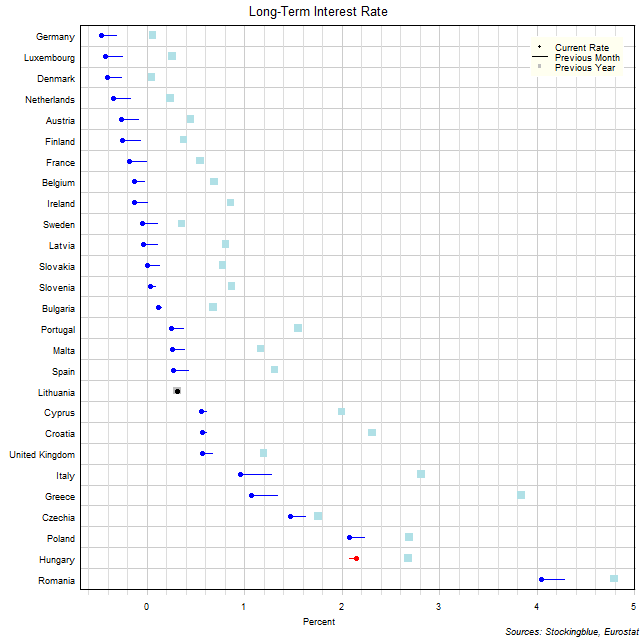The chart above shows the ten-year interest rate in each EU state as of February 2020, the change from the previous month, and the rate one year prior.  Eleven states have a negative interest rate (up from eight last month and up from zero last year).

# EU Long-Term Interest Rates, January 2020

Mar 5, 2020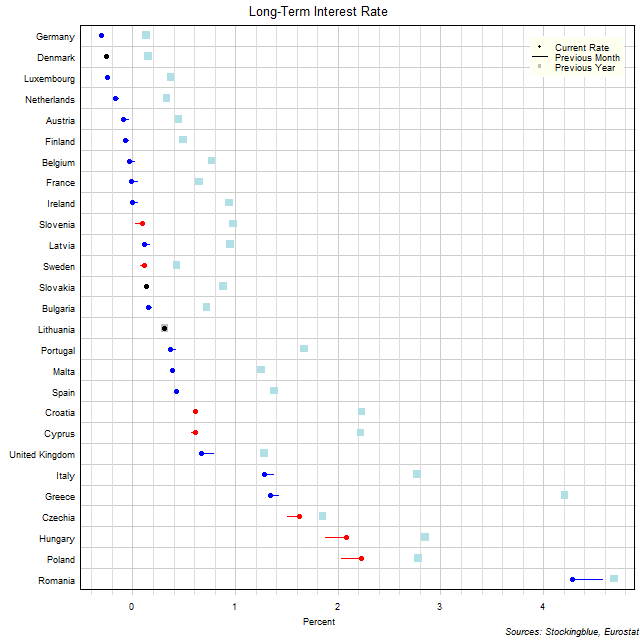The chart above shows the ten-year interest rate in each EU state as of January 2020, the change from the previous month, and the rate one year prior.  Eight states have a negative interest rate (up from six last month and up from zero last year).